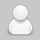﻿ 纺织基础知识大全 纺织单位选择换算公式_徐果萍博客

# 纺织基础知识 纺织单位选择换算公式## 纺织单位选择换算公式

(1)、公制支数(N)与旦尼尔(D)的换算公式：D=9000/N

(2)、英制支数(S)与旦尼尔(D)的换算公式：D=5315/S

(3)、分特克斯(dtex)与特克斯(tex)的换算公式：1tex=10dtex

(4)、特克斯(tex)与旦尼尔(D)的换算公式：tex=D/9

(5)、特克斯(tex)与英制支数(S)的换算公式：tex=K/SK值：纯棉纱K=583.1纯化纤K=590.5涤棉纱K=587.6棉粘纱(75：25)K=584.8维棉纱(50：50)K=587.0

(6)、特克斯(tex)与公制数(N)的换算公式：tex=1000/N

(7)、分特克斯(dtex)与旦尼尔(D)的换算公式：dtex=10D/9

(8)、分特克斯(dtex)与英制支数(S)的换算公式：dtex=10K/SK值：纯棉纱K=583.1纯化纤K=590.5涤棉纱K=587.6棉粘纱(75：25)K=584.8维棉纱(50：50)K=587.0

(9)、分特克斯(dtex)与公制支数(N)的换算公式：dtex=10000/N

(10)、公制厘米(cm)与英制英寸(inch)的换算公式：1inch=2.54cm

(11)、公制米(M)与英制码(yd)的换算公式：1码=0.9144米

(12)、绸缎平方米克重(g/m2)与姆米(m/m)的换算公式：1m/m=4.3056g/m2

(13)、绸缎的实际重量与磅重的换算公式：磅重(lb)=每米绸重(g/m)*0.9144(m/yd)*50(yd)/453.6(g/yd)

## 相关阅读

• 纺织基础知识 纺织织造六个工艺流程
• 纺织基础知识 纺织纺纱六个工艺流程
• 纺织基础知识 纺织面料四种分类方法
• 纺织基础知识 纺织原料六个常用概念
• 纺织基础知识 纺织原料五种检测方法
• 纺织基础知识 纺织单位选择换算公式
• 纺织基础知识 定长制定重制计算公式
• 本文来源于网络，不代表徐果萍博客立场，转载请注明出处：http://xuguoping.net/zhichang/fangzhidanwei-huansuangongshi.html#### 作者: 徐果萍

1983年5月8日出生，浙江金华兰溪人，新闻传播专业毕业，80后站长。前期十余年主要从事网站编辑以及网站运营岗位；后跨界转至仓库管理员，现任仓库主管一职。著有：文集《孤寂的执着》，小说《网站之殇》。爱好折腾网站和撰写文章。

发表评论:

◎欢迎参与讨论，请在这里发表您的看法、交流您的观点。

关注微博